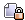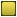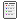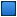###Author Topic: trucks  (Read 3945 times)

####jem7650

• Newbie
•• Posts: 10##### trucks
« on: September 02, 2018, 04:14:12 AM »
Have we implemented trucks for moving raw material? I can't move material from mine to smelter.

####leeor_net

• Hero Member
••• Posts: 2348##### Re: trucks
« Reply #1 on: September 03, 2018, 10:05:24 PM »
For now it happens automatically.

####jem7650

• Newbie
•• Posts: 10##### Re: trucks
« Reply #2 on: September 04, 2018, 03:20:52 AM »
No minerals are moved from mine to smelter. I'm I doing something wrong?

####leeor_net

• Hero Member
••• Posts: 2348##### Re: trucks
« Reply #3 on: September 04, 2018, 09:11:36 PM »
Minerals only? You're being a little too broad. There are four mined resources, all ore: Common and Rare Metal Ore and Common and Rare Mineral Ore. Is only one of these not moving or none of them?

If you can attach a save file where this is occurring it would be helpful.

####jem7650

• Newbie
•• Posts: 10##### Re: trucks
« Reply #4 on: September 05, 2018, 03:19:34 AM »
Code: [Select]
``<?xml version="1.0"?>-<OutpostHD_SaveGame version="0.30"><properties diggingdepth="4" tset="tsets/mars.png" sitemap="maps/mars_04"/><view_parameters viewlocation_y="11" viewlocation_x="215" currentdepth="0"/>-<mines><mine flags="001111" yield="1" active="0" depth="0" y="140" x="223"/><mine flags="001111" yield="1" active="0" depth="0" y="59" x="229"/><mine flags="001111" yield="2" active="0" depth="0" y="23" x="50"/><mine flags="001111" yield="1" active="0" depth="0" y="107" x="111"/><mine flags="001111" yield="1" active="0" depth="0" y="127" x="27"/><mine flags="001111" yield="1" active="0" depth="0" y="63" x="25"/><mine flags="001111" yield="1" active="0" depth="0" y="64" x="7"/><mine flags="001111" yield="1" active="0" depth="0" y="132" x="237"/><mine flags="001111" yield="0" active="0" depth="0" y="93" x="270"/><mine flags="001111" yield="1" active="0" depth="0" y="54" x="234"/><mine flags="001111" yield="0" active="0" depth="0" y="80" x="124"/><mine flags="001111" yield="1" active="0" depth="0" y="114" x="271"/><mine flags="001111" yield="2" active="0" depth="0" y="110" x="207"/>-<mine flags="010000" yield="1" active="1" depth="2" y="20" x="223"><vein rare_minerals="540" rare_metals="690" common_minerals="540" common_metals="690" id="0"/><vein rare_minerals="550" rare_metals="700" common_minerals="550" common_metals="700" id="1"/></mine><mine flags="001111" yield="1" active="0" depth="0" y="79" x="131"/><mine flags="001111" yield="2" active="0" depth="0" y="76" x="48"/><mine flags="001111" yield="0" active="0" depth="0" y="123" x="205"/><mine flags="001111" yield="1" active="0" depth="0" y="5" x="145"/><mine flags="001111" yield="1" active="0" depth="0" y="82" x="165"/><mine flags="001111" yield="1" active="0" depth="0" y="140" x="244"/><mine flags="001111" yield="1" active="0" depth="0" y="89" x="98"/><mine flags="001111" yield="1" active="0" depth="0" y="98" x="292"/><mine flags="001111" yield="0" active="0" depth="0" y="112" x="230"/><mine flags="001111" yield="1" active="0" depth="0" y="47" x="263"/><mine flags="001111" yield="2" active="0" depth="0" y="121" x="218"/><mine flags="001111" yield="1" active="0" depth="0" y="5" x="203"/><mine flags="001111" yield="1" active="0" depth="0" y="24" x="76"/><mine flags="001111" yield="1" active="0" depth="0" y="40" x="141"/><mine flags="001111" yield="1" active="0" depth="0" y="103" x="40"/><mine flags="001111" yield="0" active="0" depth="0" y="119" x="65"/></mines>-<tiles><tile depth="0" y="13" x="219" index="0"/><tile depth="0" y="14" x="219" index="0"/><tile depth="0" y="15" x="219" index="0"/><tile depth="0" y="16" x="219" index="0"/><tile depth="0" y="17" x="219" index="0"/><tile depth="0" y="12" x="221" index="0"/><tile depth="0" y="13" x="221" index="0"/><tile depth="0" y="14" x="221" index="0"/><tile depth="0" y="15" x="221" index="0"/><tile depth="0" y="16" x="221" index="0"/><tile depth="1" y="15" x="219" index="1"/><tile depth="1" y="16" x="219" index="1"/><tile depth="1" y="17" x="219" index="1"/><tile depth="1" y="18" x="219" index="1"/><tile depth="1" y="19" x="219" index="1"/><tile depth="1" y="20" x="219" index="1"/><tile depth="1" y="21" x="219" index="0"/><tile depth="1" y="22" x="219" index="1"/><tile depth="1" y="23" x="219" index="1"/><tile depth="1" y="15" x="220" index="1"/><tile depth="1" y="16" x="220" index="1"/><tile depth="1" y="17" x="220" index="1"/><tile depth="1" y="18" x="220" index="1"/><tile depth="1" y="19" x="220" index="1"/><tile depth="1" y="23" x="220" index="1"/><tile depth="1" y="15" x="221" index="1"/><tile depth="1" y="16" x="221" index="1"/><tile depth="1" y="17" x="221" index="1"/><tile depth="1" y="18" x="221" index="1"/><tile depth="1" y="19" x="221" index="1"/><tile depth="1" y="20" x="221" index="0"/><tile depth="1" y="21" x="221" index="0"/><tile depth="1" y="22" x="221" index="1"/><tile depth="1" y="23" x="221" index="1"/></tiles>-<structures><structure depth="0" y="17" x="220" id="10390320" direction="1" type="Tube" forced_idle="0" state="0" age="0"/><structure depth="0" y="12" x="220" id="59518040" direction="1" type="Tube" forced_idle="0" state="0" age="0"/><structure depth="0" y="14" x="220" id="59518440" direction="1" type="Tube" forced_idle="0" state="0" age="0"/><structure depth="0" y="20" x="220" id="67805616" direction="1" type="SEED Lander" forced_idle="0" state="1" age="30"/><structure depth="2" y="20" x="223" id="68095184" direction="1" type="Mine Shaft" forced_idle="0" state="1" age="6"/><structure depth="0" y="20" x="219" id="62714640" direction="1" type="Tube" forced_idle="0" state="0" age="0"/><structure depth="0" y="18" x="220" id="62808896" direction="1" type="Tube" forced_idle="0" state="0" age="0"/><structure depth="0" y="20" x="218" id="66880960" direction="1" type="Cargo Lander" forced_idle="0" state="1" age="24"/><structure depth="0" y="20" x="221" id="62849224" direction="1" type="Tube" forced_idle="0" state="0" age="0"/><structure depth="0" y="17" x="221" id="68096000" direction="1" type="Solar Panel Array" forced_idle="0" state="1" age="6"/><structure depth="1" y="20" x="220" id="63024064" direction="1" type="Tube" forced_idle="0" state="0" age="0"/><structure depth="0" y="13" x="220" id="63298008" direction="1" type="Tube" forced_idle="0" state="0" age="0"/><structure depth="1" y="21" x="220" id="63298408" direction="1" type="Tube" forced_idle="0" state="0" age="0"/><structure depth="0" y="18" x="221" id="19349840" direction="1" type="Storage Tanks" forced_idle="0" state="1" age="23"/><structure depth="0" y="21" x="218" id="68970624" direction="1" type="Cargo Lander" forced_idle="0" state="1" age="24"/><structure depth="0" y="20" x="223" id="164909200" direction="1" type="Mine Facility" forced_idle="0" state="1" age="23"/><structure depth="0" y="21" x="222" id="19057384" direction="1" type="Colonist Lander" forced_idle="0" state="1" age="24"/><structure depth="0" y="19" x="220" id="64560536" direction="1" type="Tube" forced_idle="0" state="0" age="0"/><structure depth="0" y="19" x="219" id="69070912" direction="1" type="SEED Power" forced_idle="0" state="1" age="30"/><structure depth="0" y="22" x="220" id="68971032" direction="4" type="Air Shaft" forced_idle="0" state="0" age="0"/><structure depth="1" y="20" x="223" id="164909616" direction="1" type="Mine Shaft" forced_idle="0" state="1" age="23"/><structure depth="0" y="18" x="219" id="165601232" direction="1" type="Agricultural Dome" forced_idle="0" state="3" age="3"/><structure depth="0" y="16" x="220" id="64562576" direction="1" type="Tube" forced_idle="0" state="0" age="0"/><structure depth="0" y="19" x="221" id="169043840" direction="1" type="Command Center" forced_idle="0" state="1" age="27"/><structure depth="0" y="15" x="220" id="64563392" direction="1" type="Tube" forced_idle="0" state="0" age="0"/><structure depth="0" y="20" x="222" id="64563800" direction="2" type="Tube" forced_idle="0" state="0" age="0"/>-<structure depth="0" y="21" x="221" id="67079744" direction="1" type="SEED Smelter" forced_idle="0" state="1" age="29"><production food="0" energy="0" rare_mineral="0" rare_metal="0" common_mineral="0" common_metal="0" rare_mineral_ore="10" rare_metal_ore="10" common_mineral_ore="10" common_metal_ore="10"/></structure><structure depth="0" y="21" x="220" id="65511632" direction="1" type="Tube" forced_idle="0" state="0" age="0"/><structure depth="0" y="21" x="219" id="66555088" direction="1" type="SEED Factory" forced_idle="0" state="1" age="30" production_type="-1" production_completed="0"/><structure depth="1" y="22" x="220" id="66870360" direction="4" type="Air Shaft" forced_idle="0" state="0" age="0"/></structures>-<robots id_counter="3"><robot depth="0" y="11" x="220" id="2" direction="1" type="1" age="29" production="1"/><robot depth="0" y="12" x="219" id="1" type="2" age="26" production="1"/></robots><resources food="21" energy="64" rare_mineral="27" rare_metal="14" common_mineral="20" common_metal="10" rare_mineral_ore="0" rare_metal_ore="0" common_mineral_ore="0" common_metal_ore="0"/><turns count="30"/><population retired="0" scientists="39" workers="40" students="19" children="0" cargo_landers="0" colonist_landers="0" prev_morale="279" morale="273"/><ai gender="0"/></OutpostHD_SaveGame>``
« Last Edit: September 05, 2018, 12:09:40 PM by leeor_net »

####leeor_net

• Hero Member
••• Posts: 2348##### Re: trucks
« Reply #5 on: September 05, 2018, 12:09:24 PM »
This works. Thank you, I'll check it out when I get home.

For the future, attaching the file vs copy/pasting its contents would be better.####leeor_net

• Hero Member
••• Posts: 2348##### Re: trucks
« Reply #6 on: September 05, 2018, 06:13:22 PM »
Okay, I see your problem. Your mine is set to not produce anything. When you right click on a mine, the Mine Production window is brought up. In your case, all of the ore production buttons are unset so the mine will basically just sit there doing nothing.

I admit that the feedback is a little lacking. I was too lazy to make checkboxes so I used toggle buttons instead.

They're toggled when they're dark, and untoggled when they're light:In future updates I'll probably switch those over to check boxes to remove any ambiguity.
« Last Edit: September 05, 2018, 06:19:47 PM by leeor_net »

####jem7650

• Newbie
•• Posts: 10##### Re: trucks
« Reply #7 on: September 06, 2018, 03:21:14 AM »
Thank you. I'll give it a shot.